# RS Aggarwal Solutions for Class 6 Chapter 8 Algebraic Expressions Exercise 8B

RS Aggarwal Solutions for Class 6 Chapter 8 Algebraic Expressions Exercise 8B PDF are given here to help students boost their exam preparation. This exercise has questions related to finding the value of Algebraic Expressions, coefficients, constant terms, like and unlike terms, etc. A combination of constants and variables connected by any one or more of the symbols is called an algebraic expression. RS Aggarwal Solutions creates interest among students in solving problems according to the current CBSE syllabus.

## Download PDF of RS Aggarwal Solutions for Class 6 Chapter 8 Algebraic Expressions Exercise 8B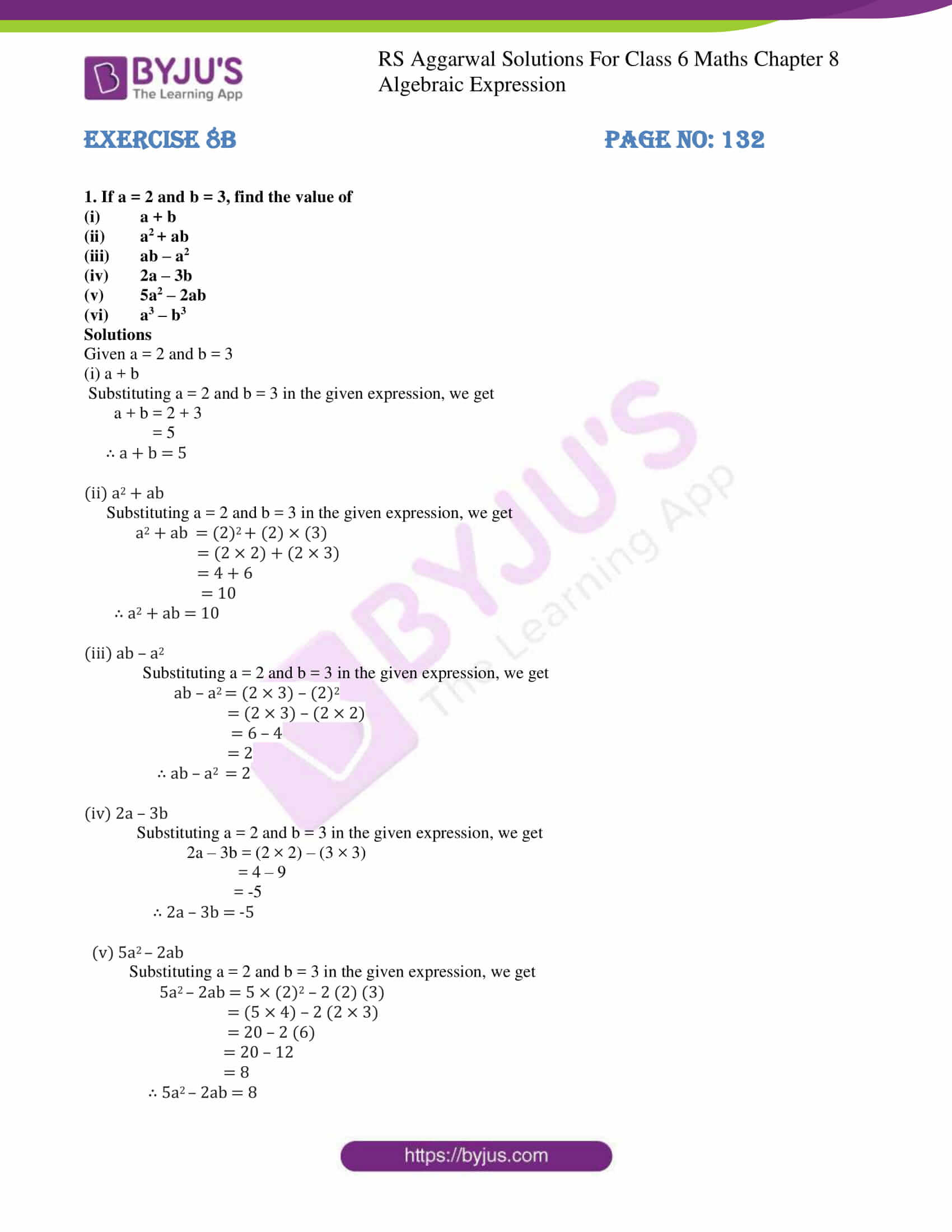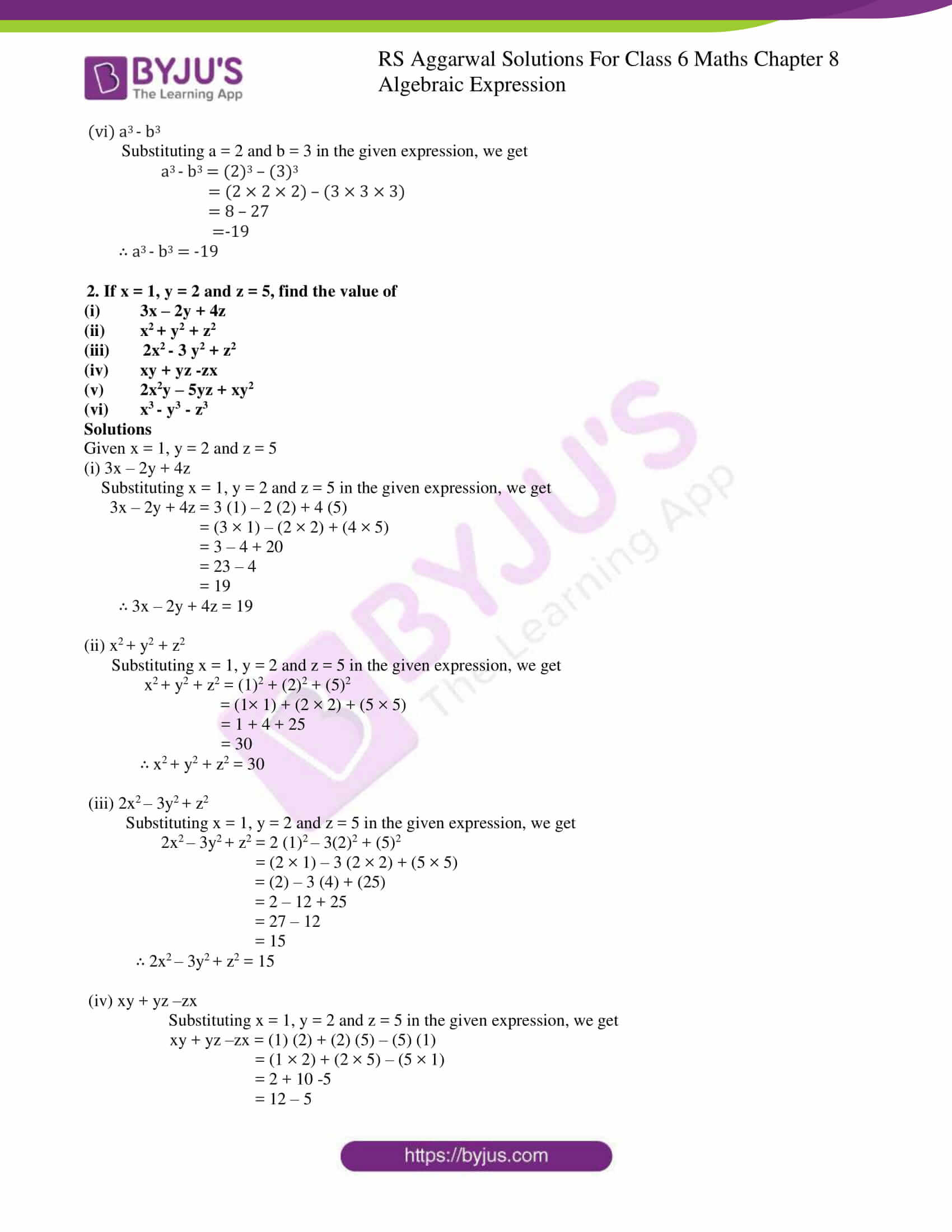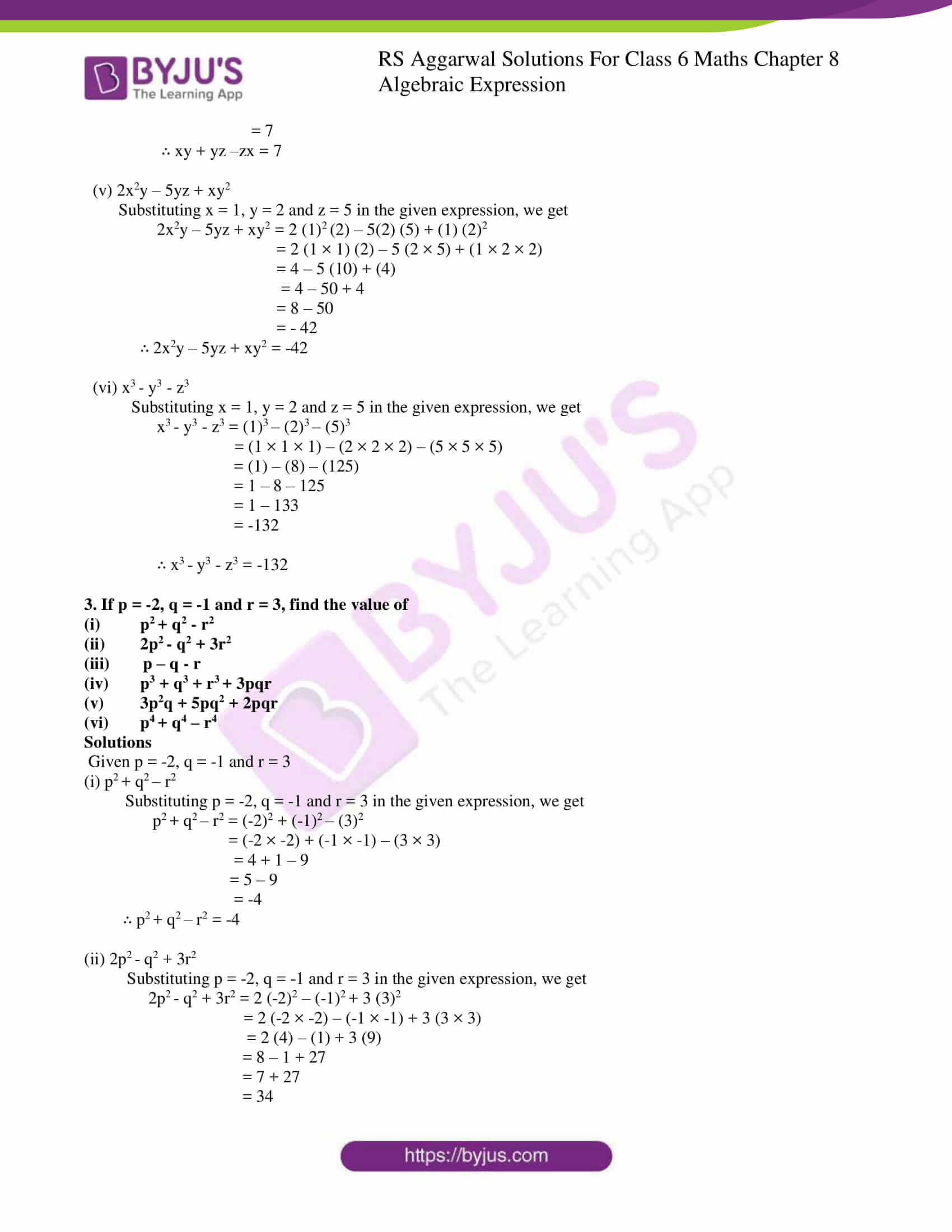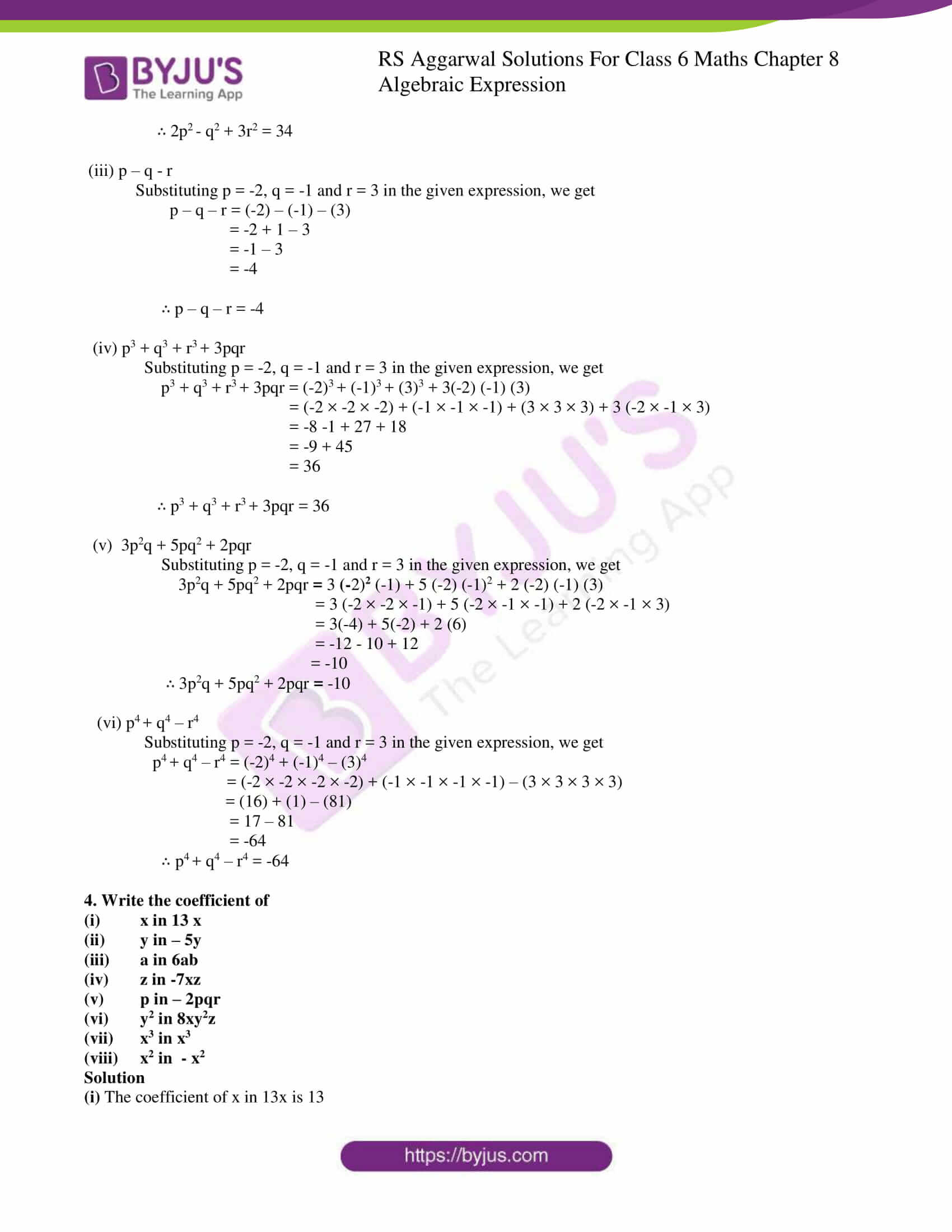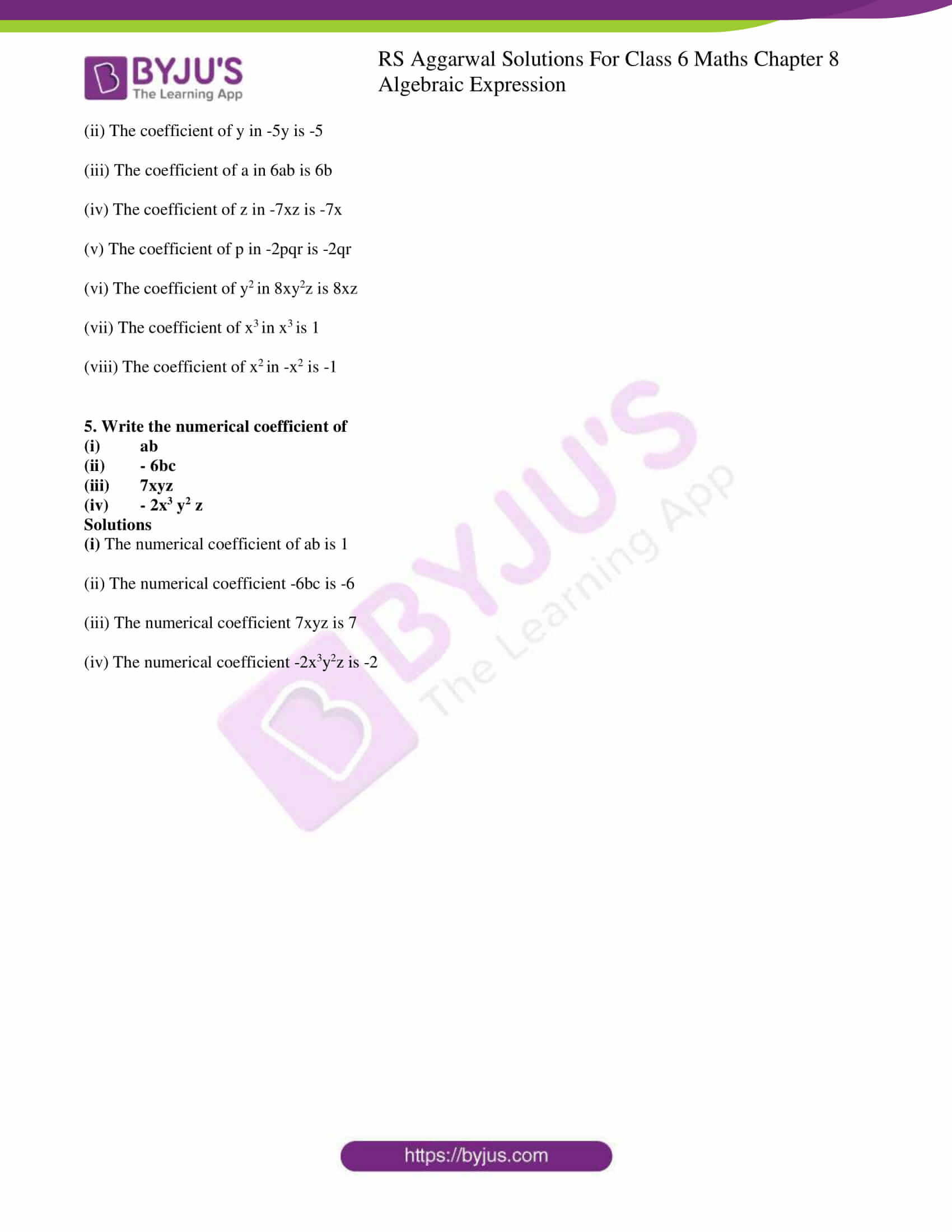### Access answers to Maths RS Aggarwal Solutions for Class 6 Chapter 8 Algebraic Expressions Exercise 8B

1. If a = 2 and b = 3, find the value of

(i) a + b

(ii) a2 + ab

(iii) ab – a2

(iv) 2a – 3b

(v) 5a2 – 2ab

(vi) a3 – b3

Solutions

Given a = 2 and b = 3

(i) a + b

Substituting a = 2 and b = 3 in the given expression, we get

a + b = 2 + 3

= 5

∴ a + b = 5

(ii) a2 + ab

Substituting a = 2 and b = 3 in the given expression, we get

a2 + ab = (2)2 + (2) × (3)

= (2 × 2) + (2 × 3)

= 4 + 6

= 10

∴ a2 + ab = 10

(iii) ab – a2

Substituting a = 2 and b = 3 in the given expression, we get

ab – a2 = (2 × 3) – (2)2

= (2 × 3) – (2 × 2)

= 6 – 4

= 2

∴ ab – a2 = 2

(iv) 2a – 3b

Substituting a = 2 and b = 3 in the given expression, we get

2a – 3b = (2 × 2) – (3 × 3)

= 4 – 9

= -5

∴ 2a – 3b = -5

(v) 5a2 – 2ab

Substituting a = 2 and b = 3 in the given expression, we get

5a2 – 2ab = 5 × (2)2 – 2 (2) (3)

= (5 4) – 2 (2 × 3)

= 20 – 2 (6)

= 20 – 12

= 8

∴ 5a2 – 2ab = 8

(vi) a3 – b3

Substituting a = 2 and b = 3 in the given expression, we get

a3 – b3 = (2)3 – (3)3

= (2 × 2 × 2) – (3 × 3 × 3)

= 8 – 27

=-19

∴ a3 – b3 = -19

2. If x = 1, y = 2 and z = 5, find the value of

(i) 3x – 2y + 4z

(ii) x2 + y2 + z2

(iii) 2x2 – 3 y2 + z2

(iv) xy + yz -zx

(v) 2x2y – 5yz + xy2

(vi) x3 – y3 – z3

Solutions

Given x = 1, y = 2 and z = 5

(i) 3x – 2y + 4z

Substituting x = 1, y = 2 and z = 5 in the given expression, we get

3x – 2y + 4z = 3 (1) – 2 (2) + 4 (5)

= (3 × 1) – (2 × 2) + (4 × 5)

= 3 – 4 + 20

= 23 – 4

= 19

∴ 3x – 2y + 4z = 19

(ii) x2 + y2 + z2

Substituting x = 1, y = 2 and z = 5 in the given expression, we get

x2 + y2 + z2 = (1)2 + (2)2 + (5)2

= (1× 1) + (2 × 2) + (5 × 5)

= 1 + 4 + 25

= 30

∴ x2 + y2 + z2 = 30

(iii) 2x2 – 3y2 + z2

Substituting x = 1, y = 2 and z = 5 in the given expression, we get

2x2 – 3y2 + z2 = 2 (1)2 – 3(2)2 + (5)2

= (2 × 1) – 3 (2 × 2) + (5 × 5)

= (2) – 3 (4) + (25)

= 2 – 12 + 25

= 27 – 12

= 15

∴ 2x2 – 3y2 + z2 = 15

(iv) xy + yz –zx

Substituting x = 1, y = 2 and z = 5 in the given expression, we get

xy + yz –zx = (1) (2) + (2) (5) – (5) (1)

= (1 × 2) + (2 × 5) – (5 × 1)

= 2 + 10 -5

= 12 – 5

= 7

∴ xy + yz –zx = 7

(v) 2x2y – 5yz + xy2

Substituting x = 1, y = 2 and z = 5 in the given expression, we get

2x2y – 5yz + xy2 = 2 (1)2 (2) – 5(2) (5) + (1) (2)2

= 2 (1 × 1) (2) – 5 (2 × 5) + (1 × 2 × 2)

= 4 – 5 (10) + (4)

= 4 – 50 + 4

= 8 – 50

= – 42

∴ 2x2y – 5yz + xy2 = -42

(vi) x3 – y3 – z3

Substituting x = 1, y = 2 and z = 5 in the given expression, we get

x3 – y3 – z3 = (1)3 – (2)3 – (5)3

= (1 × 1 × 1) – (2 × 2 × 2) – (5 × 5 × 5)

= (1) – (8) – (125)

= 1 – 8 – 125

= 1 – 133

= -132

∴ x3 – y3 – z3 = -132

3. If p = -2, q = -1 and r = 3, find the value of

(i) p2 + q2 – r2

(ii) 2p2 – q2 + 3r2

(iii) p – q – r

(iv) p3 + q3 + r3 + 3pqr

(v) 3p2q + 5pq2 + 2pqr

(vi) p4 + q4 – r4

Solutions

Given p = -2, q = -1 and r = 3

(i) p2 + q2 – r2

Substituting p = -2, q = -1 and r = 3 in the given expression, we get

p2 + q2 – r2 = (-2)2 + (-1)2 – (3)2

= (-2 × -2) + (-1 × -1) – (3 × 3)

= 4 + 1 – 9

= 5 – 9

= -4

∴ p2 + q2 – r2 = -4

(ii) 2p2 – q2 + 3r2

Substituting p = -2, q = -1 and r = 3 in the given expression, we get

2p2 – q2 + 3r2 = 2 (-2)2 – (-1)2 + 3 (3)2

= 2 (-2 × -2) – (-1 × -1) + 3 (3 × 3)

= 2 (4) – (1) + 3 (9)

= 8 – 1 + 27

= 7 + 27

= 34

∴ 2p2 – q2 + 3r2 = 34

(iii) p – q – r

Substituting p = -2, q = -1 and r = 3 in the given expression, we get

p – q – r = (-2) – (-1) – (3)

= -2 + 1 – 3

= -1 – 3

= -4

∴ p – q – r = -4

(iv) p3 + q3 + r3 + 3pqr

Substituting p = -2, q = -1 and r = 3 in the given expression, we get

p3 + q3 + r3 + 3pqr = (-2)3 + (-1)3 + (3)3 + 3(-2) (-1) (3)

= (-2 × -2 × -2) + (-1 × -1 × -1) + (3 × 3 × 3) + 3 (-2 × -1 × 3)

= -8 -1 + 27 + 18

= -9 + 45

= 36

∴ p3 + q3 + r3 + 3pqr = 36

(v) 3p2q + 5pq2 + 2pqr

Substituting p = -2, q = -1 and r = 3 in the given expression, we get

3p2q + 5pq2 + 2pqr = 3 (-2)2 (-1) + 5 (-2) (-1)2 + 2 (-2) (-1) (3)

= 3 (-2 × -2 × -1) + 5 (-2 × -1 × -1) + 2 (-2 × -1 × 3)

= 3(-4) + 5(-2) + 2 (6)

= -12 – 10 + 12

= -10

∴ 3p2q + 5pq2 + 2pqr = -10

(vi) p4 + q4 – r4

Substituting p = -2, q = -1 and r = 3 in the given expression, we get

p4 + q4 – r4 = (-2)4 + (-1)4 – (3)4

= (-2 × -2 × -2 × -2) + (-1 × -1 × -1 × -1) – (3 × 3 × 3 × 3)

= (16) + (1) – (81)

= 17 – 81

= -64

∴ p4 + q4 – r4 = -64

4. Write the coefficient of

(i) x in 13 x

(ii) y in – 5y

(iii) a in 6ab

(iv) z in -7xz

(v) p in – 2pqr

(vi) y2 in 8xy2z

(vii) x3 in x3

(viii) x2 in – x2

Solution

(i) The coefficient of x in 13x is 13

(ii) The coefficient of y in -5y is -5

(iii) The coefficient of a in 6ab is 6b

(iv) The coefficient of z in -7xz is -7x

(v) The coefficient of p in -2pqr is -2qr

(vi) The coefficient of y2 in 8xy2z is 8xz

(vii) The coefficient of x3 in x3 is 1

(viii) The coefficient of x2 in -x2 is -1

5. Write the numerical coefficient of

(i) ab

(ii) – 6bc

(iii) 7xyz

(iv) – 2x3 y2 z

Solutions

(i) The numerical coefficient of ab is 1

(ii) The numerical coefficient -6bc is -6

(iii) The numerical coefficient 7xyz is 7

(iv) The numerical coefficient -2x3y2z is -2